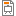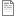# 17ABBPMS - Probability and Mathematical Statistics

Code Completion Credits Range Language
17ABBPMS Z,ZK 4 2P+2C English
Linear Algebra and Differential Calculus (17ABBLAD)
Garant předmětu:
Lecturer:
Tutor:
Supervisor:
Department of Biomedical Technology
Synopsis:

Introduction to probability theory and mathematical statistics. Determinism and chance. Axiomatic definition. Random variable and its distribution function. Discrete and continuous distributions. Quintiles. Random vectors. Conditioning and independence. Functions of random variables. Characteristics of random variables, weak law of large numbers. The role of mathematical statistics, the population and sample. Random selection. Point and interval estimates. Hypothesis testing. Goodness. Non-parametric tests.

Requirements:

Testimonial:

1) Three small tests during the semester (typical examples), tests together must be passed with at least 75 %.

2) Active participation in seminars, doing homework.

3) Allowed to be absent 3 times during the semester.

Exam:

Only students that have got testimonial and who have already passed the course 17ABBLAD (prerequisity).

Syllabus of lectures:

1. Motivational lecture. Determinism and randomness.

2. Random variable and distribution function.

3. Discrete distributions.

4. Continuous distributions.

5. Random vectors, conditioning and independence.

6. Random vectors, characteristics, functions of random variables.

7. The role of mathematical statistics.

8. Parameter estimation.

9. Testing hypotheses in a normal distribution.

10. Non-parametric tests.

11. Analysis of variance.

12. Principles of experimental design.

Syllabus of tutorials:

1. Classical and geometric probability.

2. Combinatorial problems.

3. Discrete variables.

4. Continuous variables.

5. Variable with a normal distribution.

6. Conditional and marginal distributions.

7. Bayes' theorem.

8. Point estimate parameters.

9. Interval estimation of parameters.

10. One-sample test of hypotheses.

11. One-sample test of hypotheses about the mean value compared with an interval estimate.

12. And two-sided paired test of hypotheses about the mean value.

13. Non-parametric tests.

14. Chi-squar test of hypotheses.

Study Objective:

The objective is to familiarize students with the basic principles of modern statistics based on the (Kolmogorov) theory of probability.

Study materials:

1. Chatfield C.: Statistics for Technology, 3rd edition, Chapman and Hall, London, 1992.

2. Rogalewicz V.: Pravděpodobnost a statistika pro inženýry. Skriptum ČVUT, 2. vydání, 2007. (in Czech)

Note:
The course is a part of the following study plans:

Lectures:
AttachmentSizeLecture 1-2020 Introduction to the course623.98 KB

Recommended Probability and Statistics Ebook: http://wiki.stat.ucla.edu/socr/index.php/EBook

Exercises:
AttachmentSize2022 Seminar 1- Classical and Bayes probability108.33 KB2022 Seminar 1- Results116.5 KBAPMS_seminar_01 Probability and Combinatorics v2020100572.45 KBAPMS_seminar_02 Bayes and conditional probability v20201012 (corr results)44.69 KBAPMS_seminar_03 Binomial, Poisson and discrete distr v20201012106.74 KBAPSM_seminar_04-05 Continuous random variable v20181118 with results 118.65 KBAPMS_seminar_06 Normal distribution v20181118 with results54.21 KBAPMS_seminar_07 Random vectors v2015112435.94 KBAPMS_seminar_08_estimation_point_interval_201863 KBAPM_seminar_09_onesample_test_of_mean_and_variance_2018_with_results51 KBAPMS_seminar_10_two_sample_test_of_mean_2018_with_results48.71 KBAPMS_seminar_1150.32 KB_old_APMS_seminar_1041.2 KBNew data5 KBExam - data3 KBTables from script of assoc. prof. Rogalewicz1.73 MBUseful_formulas_for_statistics.pdf232.26 KBSummary of hypothesis tests (in Czech - English version will be distributed on the tutorial))359.92 KBGUIDEBOOK FOR STUDENTS OF (BIO)-STATISTICS801.2 KBExamTopicsSuggestions2019.pdf42.67 KB